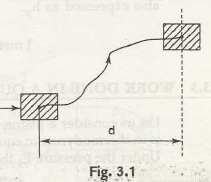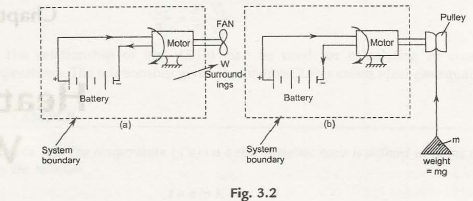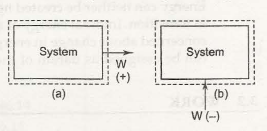When any force is applied on a body that keeps the body in move in the direction from which the force is applied is called the work was doneby force.Thus work is measurable and it is measured by the product of force and the distance covered in the direction in which theforceis applied.

Thus work, W = F.d    (f=  force, d =displacement)

The S.l unit of work = N.m or joules

Therefore 1Nm = 1J.The work done by forceover a certain distance (or of torque through an angle) is called mechanical work.

3.2.2 Thermodynamics Concept of Work

It is said to perform work when there is a change in state in the system which is meant to be a process done. There issome thethermodynamic process where force or displacement is not noticeable. For this reason, the definition of work was recreated to justify the thermal system. W work is said to be done by the system if the external factors in the system can be removed by increasing the weight against gravity. Now it is true that the net weight cannot be increased but the fact is that the external effect is reduced effectively.In the above given Fig. 3.2 (a) we see a fan driven by a motor. So here we see a system performing a work W in the surrounding. Now in the Fig. 3.2 (b) we can see that the fan is now replaced by a pulley and weight. The weight might be increased with the pulley compelled by the motor. So in this entire part, the only thing is done to increase the weight.

3.2.3 Convention of sigh of work

When the work is done by the system, then it is positive, and when work is done on a system, then it becomes negative.I the above displayed Fig. 3.3 (a) we see a positive work whereas in the Fig. 3.3 (b) the work is negative. Thus the Power is well-defined as the rate at which the system does work. Therefore the unit of power is 1J /S Ys or watt. Thus the system and surroundings can relate each other through work. So the unit of power is also conveyed as hp.

1 hP = 746 watt (British)

1 metric hp = 745.5 watt.

Links of Next Mechanical Engineering Topics:-### Customer Reviews

My Homework Help
Rated 5.0 out of 5 based on 510 customer reviews at